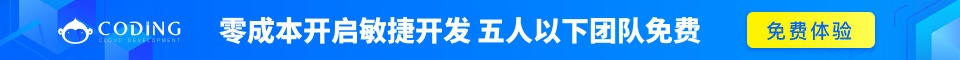# C 作用域规则

1. 在函数或块内部的局部变量
2. 在所有函数外部的全局变量
3. 形式参数的函数参数定义中

## 局部变量

```#include <stdio.h>

int main ()
{
/* 局部变量声明 */
int a, b;
int c;

/* 实际初始化 */
a = 10;
b = 20;
c = a + b;

printf ("value of a = %d, b = %d and c = %d\n", a, b, c);

return 0;
}
```

## 全局变量

```#include <stdio.h>

/* 全局变量声明 */
int g;

int main ()
{
/* 局部变量声明 */
int a, b;

/* 实际初始化 */
a = 10;
b = 20;
g = a + b;

printf ("value of a = %d, b = %d and g = %d\n", a, b, g);

return 0;
}
```

```#include <stdio.h>

/* 全局变量声明 */
int g = 20;

int main ()
{
/* 局部变量声明 */
int g = 10;

printf ("value of g = %d\n",  g);

return 0;
}
```

```value of g = 10
```

## 形式参数

```#include <stdio.h>

/* 全局变量声明 */
int a = 20;

int main ()
{
/* 在主函数中的局部变量声明 */
int a = 10;
int b = 20;
int c = 0;

printf ("value of a in main() = %d\n",  a);
c = sum( a, b);
printf ("value of c in main() = %d\n",  c);

return 0;
}

/* 添加两个整数的函数 */
int sum(int a, int b)
{
printf ("value of a in sum() = %d\n",  a);
printf ("value of b in sum() = %d\n",  b);

return a + b;
}
```

```value of a in main() = 10
value of a in sum() = 10
value of b in sum() = 20
value of c in main() = 30
```

int 0
char '\0'
float 0
double 0
pointer NULL